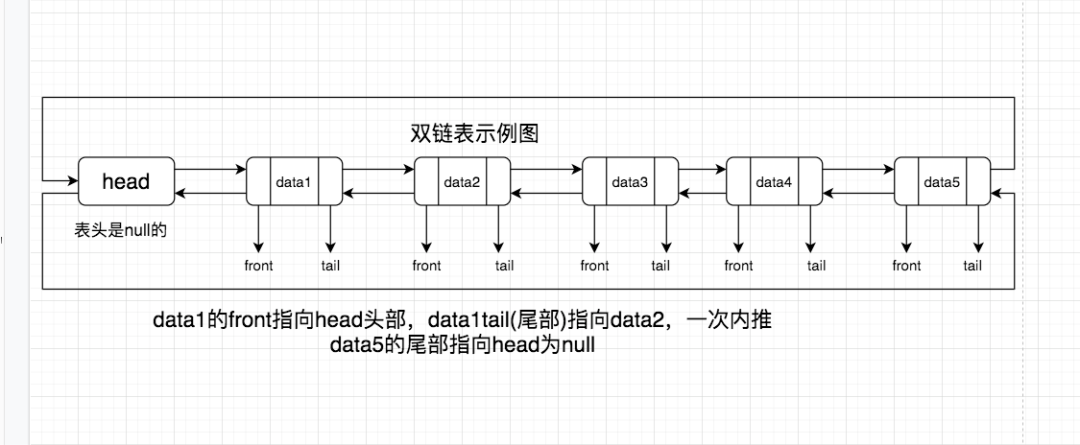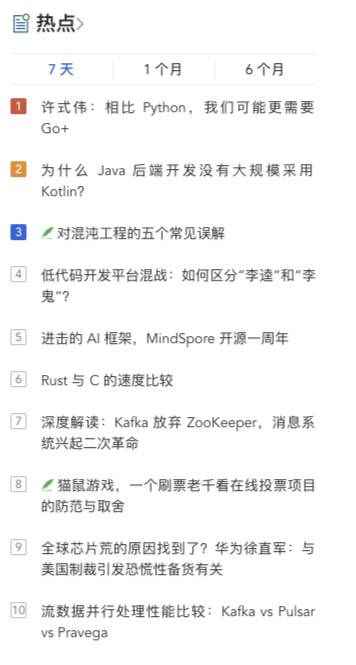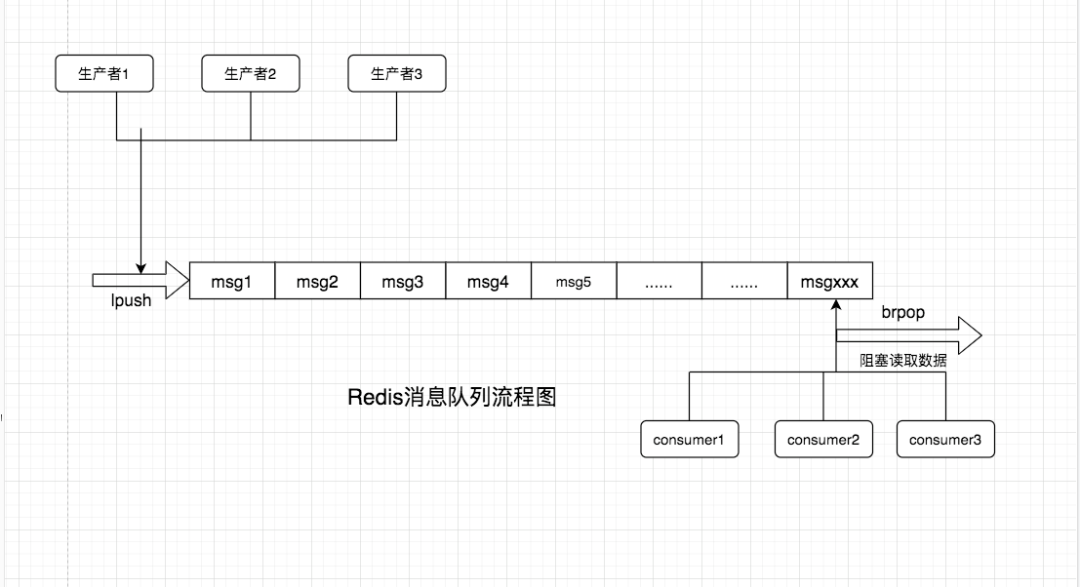# 面试系列 -2 redis 列表场景分析实践## 2 数据结构 list 的理解Redis 官方术语：列表是简单的字符串列表(说通俗点，存储的还是字符串)，按照插入顺序排序。你可以添加一个元素到列表的头部(左边)或者尾部(右边)，个列表最多可以包含^32-1 个元素(每个列表超过 40 亿个元素)。1 、数组与链表区别

2、单链表和双链表的区别

## 3 额，这才是正式问答嘛

### 3.1 列表常用命令以及注意事项

1.添加 insert 指令操作：

lpush指令：lpush key value ... 从左边插入元素(将一个或多个值插入到列表头部)；时间复杂度O(1)。rpush指令：rpush key value ... 从右边插入元素(将一个或多个值插入到列表的尾部(最右边))；时间复杂度O(1)。linsert指令：linsert key before|after pivot(指定元素) value(插入值) 向某个元素前/后插入元素，返回结果为当前列表长度，注意列表不存在或者指定元素不存在列表中时，都将不执行任何操作。时间复杂度O(n)，n为寻找指定元素中经过的元素数量。

2.查找 select 指令操作：

lrange指令：lrange key start end 获取列表中指定范围内的元素列表；若start值大于列表end值则返回空列表；时间复杂度O(s+n) s为偏移量start，n为指定区间元素数量。lindex指令：lindex key index(可负值) 获取列表指定索引下标的元素；时间复杂度O(n)，n为到达下标index过程中经过的元素数量；列表头部和尾部元素获取，时间复杂度O(1)。llen指令：llen key 获取列表长度；列表不存在则返回0，时间复杂度O(1)。

3.删除 delete 指令操作：

lpop指令：lpop key 从列表左侧弹出元素并返回头部元素；时间复杂度O(1)rpop指令：rpop key 从列表右侧弹出元素并返回尾部元素；时间复杂度O(1)lrem指令：lrem key count value 从列表中找到等于value的元素进行删除，根据count的不同分为三种情况，时间复杂度O(n)，n为列表的长度：count > 0，从表头开始向表尾，移除数量为count个元素；count < 0，从表尾开始向表头，移除数量为count的绝对值个元素；count = 0，移除表中所有与 value 相等的值；ltrim指令：ltrim key start end 按照索引范围修建列表，相当于切片操作；时间复杂度O(n)，n为被移除的元素的数量。

4.修改 update 操作：

lset指令：lset key index value 修改指定下标的元素的值设置为value；时间复杂度：① 对头元素(第一个)或尾元素(最后一个)进行操作时，复杂度为O(1)。② 其他情况下，复杂度为O(n)，n为列表的长度。

5.阻塞 block 指令操作：

blpop指令：blpop key [key ...多个key] timeout 移出并获取列表的第一个元素， 如果列表没有元素会阻塞列表直到等待超时或发现可弹出元素为止；时间复杂度O(1)。brpop指令：brpop key [key....多个key] timeout 移出并获取列表的最后一个元素， 如果列表没有元素会阻塞列表直到等待超时或发现可弹出元素为止；时间复杂度O(1)。

### 3.2.1 人气榜单+热点新闻/** * @desc 列表案例一  热点新闻 固定列表长度 维护列表长度 * @param array $data 需要加入队列的数据 * @return bool|int */public function hotNewsRank($data = array()){    if (!$data || !is_array($data)) return false;    $data = json_encode($data);    $key = sprintf('every:month:hot:news:%s', date('%Y%m')); // 每月热点新闻只展示前10条$length = $this->redis->lLen($key);    if ($length >= 10) return false;$result = $this->redis->lPush($key, $data); return$result;}

### 3.3.2 消息队列/** * @desc 队列生产者 * @param string $qname * @param array$data * @return bool */public function push($qname = '',$data = []){    if (!$qname || !$data) return false;    if (!is_array($data))$data = json_encode($data); // 进入队列$result = $this->redis->rPush($qname, $data); if ($result) return true;    // 此处可以增加一条失败队列 处理 或者记录日志 方便后续处理 或者增加告警    return false;}

/** * @desc 消息队列实现 部分代码 */while (true) {    $info =$this->redis->lPop($qname); if (!$info) break;    $data = json_encode($info, true);    if (!$data) break;$run_count = $run_count + 1;$retval = call_user_func_array($handler, [$data, $qname]); if ($retval) break;    if (time() - $start_time >$max_runtime) break;}

### 3.3.3 延迟队列

while (true) {    $info =$this->redis->lPop($qname); if (!$info) break;    $data = json_encode($info, true);    if (!$data) break;$delay_time = isset($data['delay']) ?$data['delay'] : 0;    if ($delay_time <= 0) break; if ($delay_time > time()) {        $res =$this->redis->lPush($qname,$info);        if ($res) {$length = $this->redis->lLen($qname);            if ($length > 10000) { // 队列积压严重 // todo 短信告警开发人员 处理 } else { // todo 失败落入日志文件 人为介入 或者定时检测日志文件数据 重新消费 } } break; } // 处理逻辑$run_count = $run_count + 1;$retval = call_user_func_array($handler, [$data, $qname]); if (!$retval) break;    if (time() - $start_time >$max_runtime) break;}

PS：面试官暗地里已经对你竖起了大拇指。但是他却不知道的是此刻你却竖起了中指，在椅子背后！

### 最后总结## 评论